NCERT Solutions for Class 7 Maths Chapter 1 Integers

Contents

NCERT Solutions for Class 7 Maths Chapter 1 Integers Ex 1.1

NCERT Solutions for Class 7 Maths Chapter 1 Integers Exercise 1.1
Ex 1.1 Class 7 Maths Question 1.
Following number line shows the temperature in degree Celsius (°C) at different places on a particular day.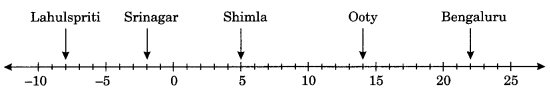(a) Observe this number line and write the temperature of the places marked on it.
(b) What is the temperature difference between the hottest and the coldest places among the above?
(c) What is the temperature difference between Lahulspriti and Srinagar?
(d) Can we say temperature of Srinagar and Shimla taken together is less than the temperature at Shimla? Is it also less than the temperature at Srinagar?
Solution:
(a) From the given number line, we observe the following temperatures.

 Cities Temperature Lahulspriti -8°C Srinagar -2°C Shimla 5°C Ooty 14°C Bengaluru 22°C

(b) The temperature of the hottest place = 22°C
The temperature of the coldest place = -8°C
Difference = 22°C – (-8°C)
= 22°C + 8°C = 30°C
(c) Temperature of Lahulspriti = -8°C
Temperature of Srinagar = -2°C
∴ Difference = -2°C – (-8°C)
= -2°C + 8°C = 6°C
(d) Temperature of Srinagar = -2°C
Temperature of Shimla = 5°C
∴ Temperature of the above cities taken together
= -2°C + 5°C = 3°C
Temperature of Shimla = 5°C
Hence, the temperature of Srinagar and Shimla taken together is less than that of Shimla by 2°C.
i.e., (5°C – 3°C) = 2°C

Ex 1.1 Class 7 Maths Question 2.
In a quiz, positive marks are given for correct answers and negative marks are given for incorrect answers. If Jack’s scores in five successive rounds were 25, -5, -10, 15 and 10, what was his total at the end?
Solution:
Given scores are 25, -5, -10, 15, 10
= 25 + 15 + 10 = 50
= (-5) + (-10) = -15
∴ Total marks given at the end
= 50 + (-15) = 50 – 15 = 35

Ex 1.1 Class 7 Maths Question 3.
At Srinagar temperature was -5°C on Monday and then it dropped by 2°C on Tuesday. What was the temperature of Srinagar on Tuesday? On Wednesday, it rose by 4°C. What was the temperature on this day?
Solution:
Initial temperature of Srinagar on Monday = -5°C
Temperature on Tuesday = -5°C – 2°C = -7°C
Temperature was increased by 4°C on Wednesday.
∴ Temperature on Wednesday
= -7°C + 4°C = -3°C
Hence, the required temperature on Tuesday = -7°C
and the temperature on Wednesday = -3°C

Ex 1.1 Class 7 Maths Question 4.
A plane is flying at the height of 5000 m above the sea level. At a particular point, it is exactly above a submarine flowing 1200 m below the sea level. What is the vertical distance between them?Solution:
Height of the flying plane = 5000 m
Depth of the submarine = -1200 m
∴ Distance between them
= + 5000 m – (-1200 m)
= 5000 m + 1200 m = 6200 m
Hence, the vertical distance = 6200 m

Ex 1.1 Class 7 Maths Question 5.
Mohan deposits ₹ 2,000 in a bank account and withdraws ₹ 1,642 from it, the next day. If withdrawal of amount from the account is represented by a negative integer, then how will you represent the amount deposited? Find the balance in Mohan’s account after the withdrawal.
Solution:
The deposited amount will be represented by a positive integer i.e., ₹ 2000.
Amount withdrawn = ₹ 1,642
∴ Balance in the account
= ₹ 2,000 – ₹ 1,642 = ₹ 358
Hence, the balance in Mohan’s account after the withdrawal
= ₹ 358

Ex 1.1 Class 7 Maths Question 6.
Rita goes 20 km towards east from a point A to the point B. From B, she moves 30 km towards west along the same road. If the distance towards east is represented by a positive integer, then how will you represent her final position from A?Solution:
Distances travelled towards east from point A will be represented by positive integer i.e. +20 km.
Distance travelled towards the west from point B will be represented by negative integer, i.e., —30 km.
Final position of Rita from A
= 20 km – 30 km = – 10 km
Hence, the required position of Rita will be presented by a negative number, i.e., -10.

Ex 1.1 Class 7 Maths Question 7.
In a magic square each row, column and the diagonal have the same sum. Check which of the following is a magic square?Solution:
(i) Row one R1 = 5 + (-1) + (—4)
=5 – 1 – 4 = 5 – 5 = 0
Row two R2 = (-5) + (-2) + 7
= -5 – 2 + 7 = -7 + 7 = 0
Row three R3 = 0 + 3 + (-3)
= 0 + 3- 3 = 0
Column one C1t = 5 + (-5) + 0
= 5 – 5 + 0 = 0
Column two C2 = (-1) + (-2) + (3)
=-1 – 2 + 3 = -3 + 3 = 0
Column three C3 = (-4) + 7 + (-3)
= -4 + 7 – 3 = 7 – 7 = 0
Diagonal d12 = 5 + (-2) + (-3)
= 5 – 2- 3 = 5 – 5 = 0
Diagonal d2 = (-4) + (-2) + 0
= -4 – 2 + 0 = -6 + 0 = -6
Here, the sum of the integers of diagonal d2 is different from the others.
Hence, it is not a magic square.

(ii) Row one R1 = 1 + (-10) + 0
= 1 – 10 + 0 = -9
Row two R2 = (-4) + (-3) + (-2)
= -4 – 3 – 2 = -9
Row three R3 = (-6) + (4) + (-7)
= -6 + 4 – 7 = -9
Column one C3 = 1 + (-4) + (-6)
= 1 – 4 – 6 = -9
Column two C2 = (-10) + (-3) + 4
= -10 – 3 + 4 = -9
Column three C3 = 0 + (-2) + (-7)
= 0 – 2 -7 = -9
Diagonal d1 = 1 + (-3) + (-7)
= 1 – 3 – 7 = 1 – 10 = -9
Diagonal d2 = 0 + (-3) + (-6)
= 0 – 3- 6 = -9
Here, sum of the integers column wise, row wise and diagonally is same i.e. -9.
Hence, (ii) is a magic square.

Ex 1.1 Class 7 Maths Question 8.
Verify a – (-b) = a + b for the following values of a and 6.
(i) a = 21, b = 18
(ii) a = 118, b = 125
(iii) a = 75, b = 84
(iv) a= 28, 6 = 11
Solution:
(i) a – (-b) = a + b
LHS = 21 – (-18) = 21 + 18 = 39
RHS = 21 + 18 = 39
LHS = RHS Hence, verified.

(ii) a – (-b) = a + b
LHS = 118 – (-125) = 118 + 125 = 243
RHS = 118 + 125 = 243
LHS = RHS Hence, verified.

(iii) a – (-b) = a + b
LHS = 75 – (-84) = 75 + 84 = 159
RHS = 75 + 84 = 159
LHS = RHS Hence, verified.

(iv) a – (-b) = a + b
LHS = 28 – (-11) = 28 + 11 = 39
RHS = 28 + 11 = 28 + 11 = 39
LHS = RHS Hence, verified.

Ex 1.1 Class 7 Maths Question 9.
Use the sign of >, < or = in the box to make the statements true.
(a) (-8) +(-4) □(-8)-(-4)
(b) (-3) + 7 – (19) □ 15 – 8 + (-9)
(c) 23 – 41 + 11 □ 23 – 41 – 11
(d) 39 + (-24) – (15) □ 36 + (-52) – (-36)
(e) -231 + 79 + 51 □ -399 + 159 + 81
Solution:
(a) (-8) + (-4) □ (-8) – (-4)
LHS = (-8) + (-4) = -8 – 4 = – 12
RHS = (-8) – (-4) = -8 + 4 = -4
Here – 12 < -4
Hence, (-8) + (-4) [<] (-8) – (-4)

(b) (-3) + 7 – (19) □ 15 – 8 + (-9)
LHS = (-3) + 7 – (19) =-3 + 7-19
= -3 – 19 + 7
= -22 + 1 = -15
RHS = 15 – 8 + (-9)
= 15-8-9
= 15 – 17 = -2
Here -15 < -2
Hence, (-3) + 7 – (19) [<] 15 – 8 + (-9)

(c) 23 – 41 + 11 □ 23 – 41 – 11
LHS = 23 – 41 + 11 = 23 + 11 – 41 = 34 – 41 = -7
RHS = 23 – 41 – 11 = 23 – 52 = -29 Here, -7 > -29
Hence, 23 – 41 + 11 [>] 23 – 41 – 11

(d) 39 + (-24) – (15) □ 36 + (-52) – (-36)
LHS = 39 + (-24) – (15)
= 39 – 24 – 15
= 39 – 39 = 0
RHS = 36 + (-52) – (-36) = 36 – 52 + 36
= 36 + 36 – 52
= 72 – 52 = 20
Here 0 < 20
Hence, 39 + (-24) – (15) [<] 36 + (-52) – (-36)

(e) -231 + 79 + 51 □ -399 + 159 + 81
LHS = -231 + 79 + 51 = -231 + 130 = -101
RHS = -399 + 159 + 81 = -399 + 240 = -159
Here, -101 > -159
Hence, -231 + 79 + 51 [>] -399 + 159 + 81

Ex 1.1 Class 7 Maths Question 10.
A water tank has steps inside it. A monkey is sitting on the topmost step (i.e., the first step). The water level is at the ninth step.
(i) He jumps 3 steps down and then jumps back 2 steps up. In how many jumps will he reach the water level?
(ii) After drinking water, he wants to go back. For this, he jumps 4 steps up and then jumps back 2 steps down in every move. In how many jumps will he reach back the top step?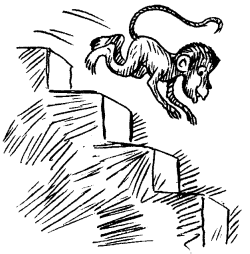(iii) If the number of steps moved down is represented by negative integers and the number of steps moved up by positive integers, represent his move in part (t) and (ii) by completing the following:
(a) – 3 + 2 – … = -8
(b) 4 – 2 + … = 8. In (a) the sum (-8) represents going down by eight steps. So, what will the sum 8 in
(b) represent?
Solution:
(i) The position of monkey after the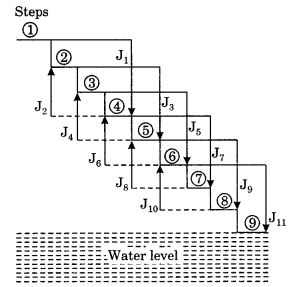1st jump J1 is at 4th step ↓
2nd jump J2 is at 2nd step ↑
3rd jump J3 is at 5th step ↓
4th jump J4 is at 3rd step ↑
5th jump J5 is at 6th step ↓
6th jump J6 is at 4th step ↑
7th jump J7 is at 7th step ↓
8th jump J8 is at 5th step ↑
9th jump J9 is at 8th step ↓
10th jump J10 is at 6th step ↑
11th jump J11 is at9th step ↓ (Water level)
Hence the required number of jumps = 11.

(ii) Monkey’s position after the1st jump J1 is at 5th step ↑
2nd jump J2 is at 7th step ↓
3rd jump J3 is at 3rd step ↑
4th jump J4 is at 5th step ↓
5th jump J5 is at 1st step ↑
Hence, the required number of jumps = 5.

(iii) According to the given conditions we have the following tables

 Jumps J1 J2 J3 J4 J5 J6 J7 J8 J9 J10 J11 Number of steps -3 +2 -3 +2 -3 +2 -3 +2 -3 +2 -3

Therefore (a) Total number of steps
=-3 + 2 – 3 + 2 – 3 + 2 – 3 + 2 – 3 + 2 – 3
= -8 which represents the monkey goes down by 8 steps.
In case (ii), we get

 Jumps J1 J2 J3 J4 J5 Number of steps + 4 -2 +4 -2 +4

Therefore (b) Total number of steps.
= +4 – 2 + 4 – 2 + 4 = 8
Here, the monkey is going up by 8 steps.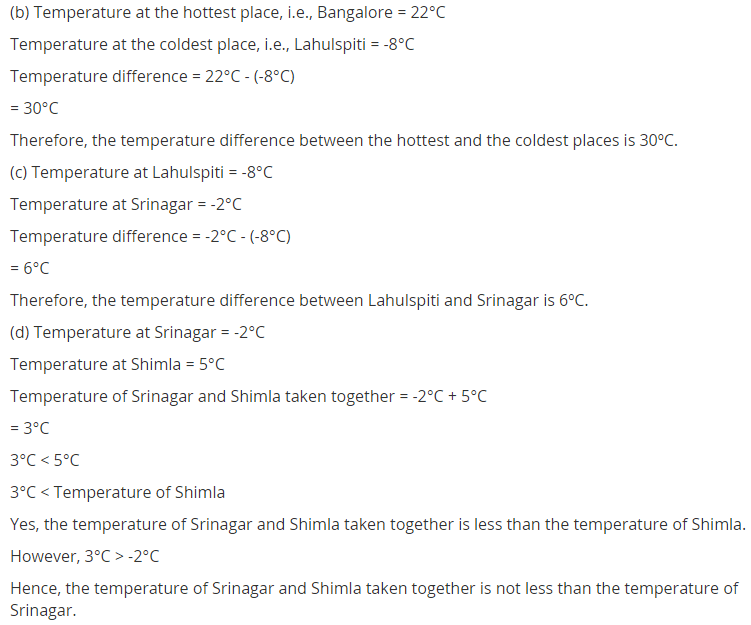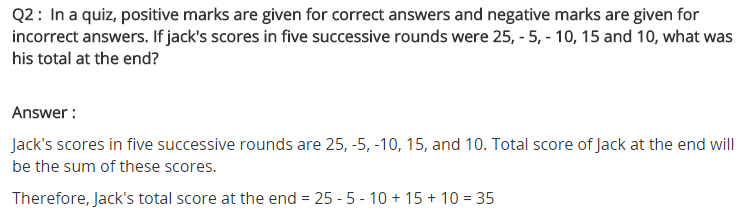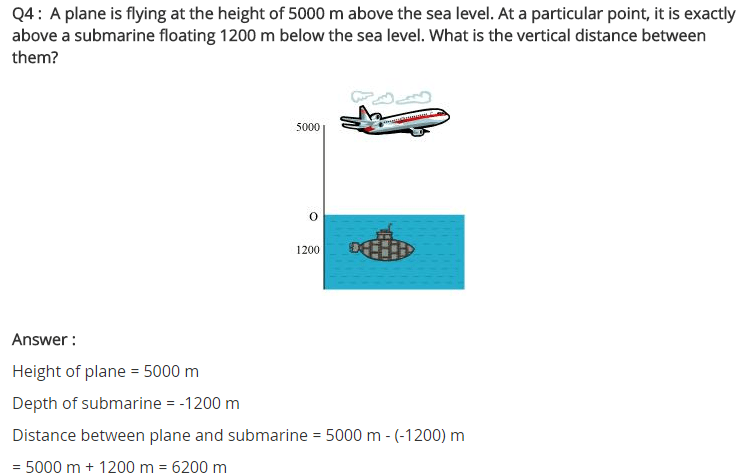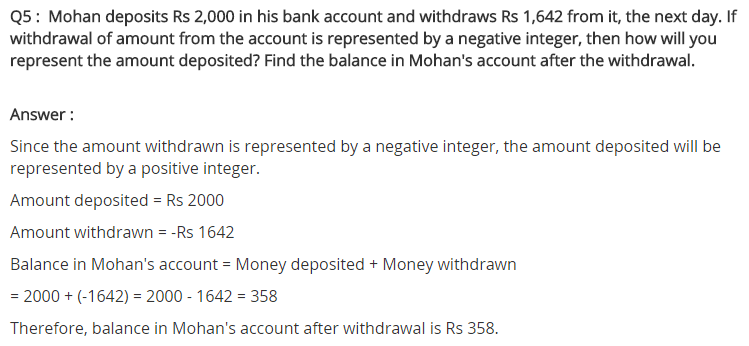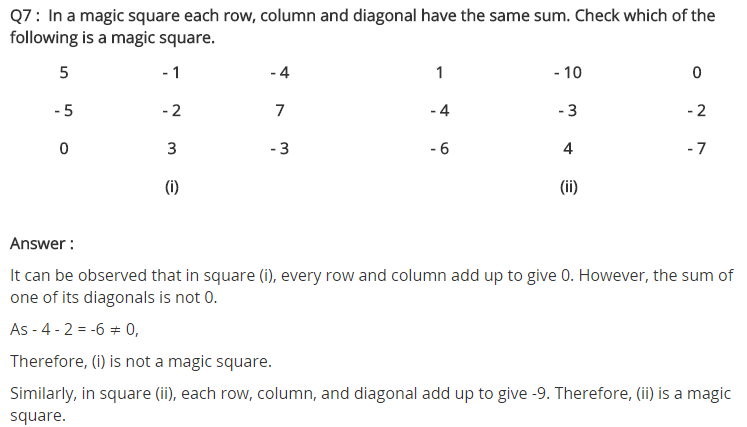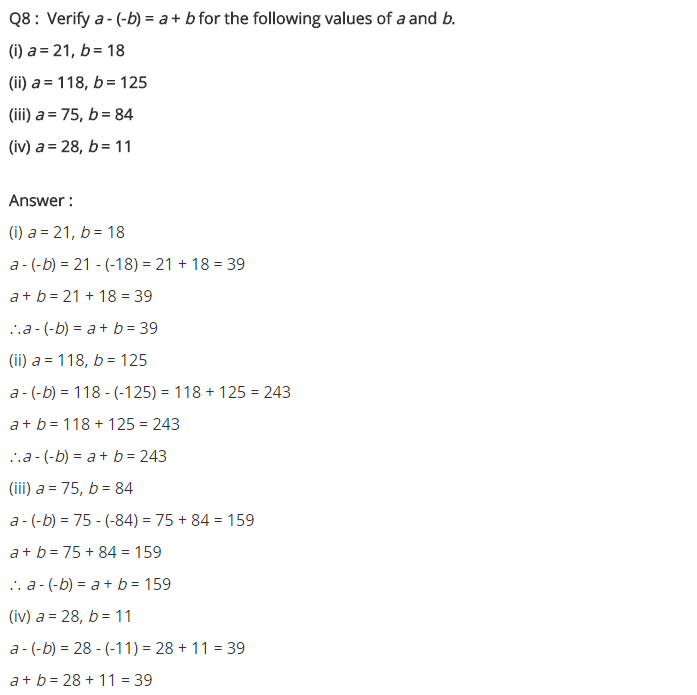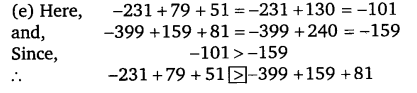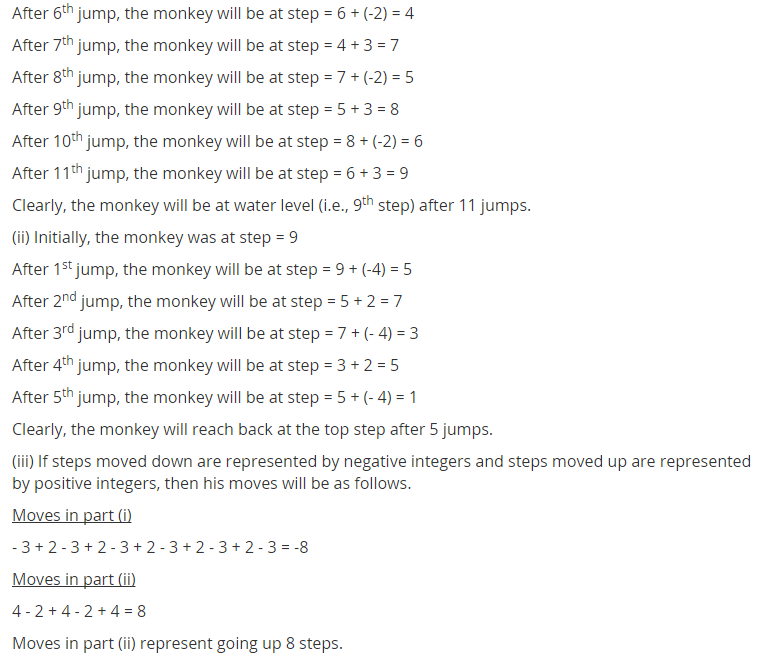NCERT Solutions